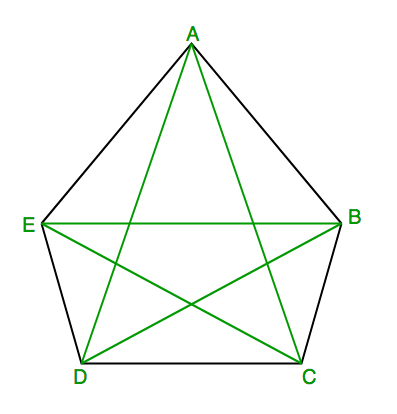# Find number of diagonals in n sided convex polygon

Given n > 3, find number of diagonals in n sided convex polygon.
According to Wikipedia, In geometry, a diagonal is a line segment joining two vertices of a polygon or polyhedron, when those vertices are not on the same edge. Informally, any sloping line is called diagonal.

Examples :

```Input : 5
Output : 5```

Explanation: Five possible diagonals are : AC, AD, BD, BE, CERecommended Practice

Since for an n-sided convex polygon, from each vertex, we can draw n-3 diagonals leaving two adjacent vertices and itself. Following this way for n-vertices, there will be n*(n-3) diagonals but then we will be calculating each diagonal twice so total number of diagonals become n*(n-3)/2
Here is code for above formula.

## C++

 `#include ` `using` `namespace` `std;`   `// C++ function to find number of diagonals` `// in n sided convex polygon` `int` `numberOfDiagonals(``int` `n)` `{` `    ``return` `n * (n - 3) / 2;` `}`   `// driver code to test above function` `int` `main()` `{` `    ``int` `n = 5;` `    ``cout << n << ``" sided convex polygon have "``;` `    ``cout << numberOfDiagonals(n) << ``" diagonals"``;` `    ``return` `0;` `}`

## Java

 `// Java function to find number of diagonals` `// in n sided convex polygon`   `public` `class` `Diagonals {`   `    ``static` `int` `numberOfDiagonals(``int` `n)` `    ``{` `        ``return` `n * (n - ``3``) / ``2``;` `    ``}`   `    ``// driver code to test above function` `    ``public` `static` `void` `main(String[] args)` `    ``{` `        ``int` `n = ``5``;` `        ``System.out.print(n + ``" sided convex polygon have "``);` `        ``System.out.println(numberOfDiagonals(n) + ``" diagonals"``);` `    ``}` `}`   `// This code is contributed by Saket Kumar`

## Python3

 `# Python3 program to find number of diagonals` `# in n sided convex polygon` `def` `numberOfDiagonals(n):` `    ``return` `n ``*` `(n ``-` `3``) ``/` `2`   ` `  `# driver code to test above function` `def` `main():` `    ``n ``=` `5` `    ``print``(n , ``" sided convex polygon have "``)` `    ``print``(numberOfDiagonals(n) , ``" diagonals"``)`   `if` `__name__ ``=``=` `'__main__'``:` `    ``main()`   `#this code contributed by 29AjayKumar`

## C#

 `// C# function to find number of diagonals` `// in n sided convex polygon` `using` `System;`   `class` `GFG {` `    `  `    ``static` `int` `numberOfDiagonals(``int` `n)` `    ``{` `        ``return` `n * (n - 3) / 2;` `    ``}`   `    ``// driver code to test above function` `    ``public` `static` `void` `Main()` `    ``{` `        ``int` `n = 5;` `        ``Console.Write(n + ``" sided convex polygon have "``);` `        `  `        ``Console.WriteLine(numberOfDiagonals(n) +` `                                  ``" diagonals"``);` `    ``}` `}`   `// This code is contributed by Sam007`

## PHP

 ``

## Javascript

 ``

Output :

`5 sided convex polygon have 5 diagonals`

Time Complexity: O(1)

Auxiliary Space: O(1)

If you like GeeksforGeeks and would like to contribute, you can also write an article using write.geeksforgeeks.org or mail your article to review-team@geeksforgeeks.org. See your article appearing on the GeeksforGeeks main page and help other Geeks.